Electron. J. Differential Equations, Vol. 2019 (2019), No. 91, pp. 1-16.

### Decay estimates for nonlinear wave equations with variable coefficients Michael Roberts

Abstract:
We study the long-time behavior of solutions to a particular class of nonlinear wave equations that appear in models for waves traveling in a non-homogeneous gas with variable damping. Specifically, decay estimates for the energy of such solutions are established. We find three different regimes of energy decay depending on the exponent of the absorption term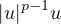and show the existence of two critical exponents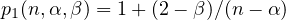and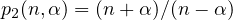. For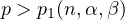, the decay of solutions of the nonlinear equation coincides with that of the corresponding linear problem. For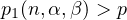, the solution decays much faster. The other critical exponent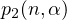further divides this region into two subregions with different decay rates. Deriving the sharp decay of solutions even for the linear problem with potential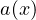is a delicate task and requires serious strengthening of the multiplier method. Here we use a modification of an approach of Todorova and Yordanov to derive the exact decay of the nonlinear equation.

Submitted March 19, 2019. Published July 23, 2019.
Math Subject Classifications: 35B33, 35B40, 35L70.
Key Words: Energy estimates; dissipative non-linear wave; subsolution; approximate solution; nonlinear exponent.

Show me the PDF file (361 KB), TEX file for this article.Michael Roberts Department of Mathematics North Dakota State University Minard 408. 1210 Albrecht Boulevard Fargo, ND 58108-6050, USA email: michael.roberts.1@ndus.edu